# Platonic Solids: Antiquity To Now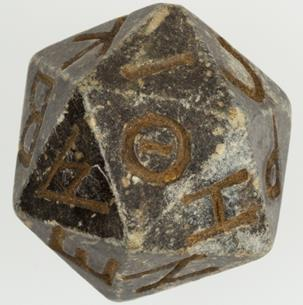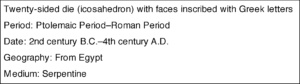Shapes have fascinated humans since the beginning of time. The polyhedra, or solid shapes with flat polygonal faces, is one of such shapes. One of the most appealing types of polyhedra is the icosahedron, most likely due to its high degree of symmetry. In the image above, you can see that the view from the center of each face, as well as the view from each corner, is precisely the same. This is what we mean by “high degree of symmetry” – many places on the polyhedron have identical views. You might notice that an icosahedron resembles something familiar: a gaming die.

As with most things, there is a functional and practical side as well. The twenty faces are symmetry-equivalent to one another, so, when the die is rolled, it has an equal chance of landing on any of the faces. An icosahedron creates what is known in the gaming world as a “fair die”.

Let’s dig a little deeper into why the icosahedron has such a high degree of symmetry. Each face is an equilateral triangle, and that there are five equilateral triangle faces meeting at every corner. That’s interesting! But, what if instead of a triangle, we use any polygon with equal sides and angles for the faces? Furthermore, what if we arrange as many of those polygonal shapes as we want at every corner (as long as we have the same number at every corner)? It turns out that there are only four more possibilities besides the icosahedron, and they are pictured below.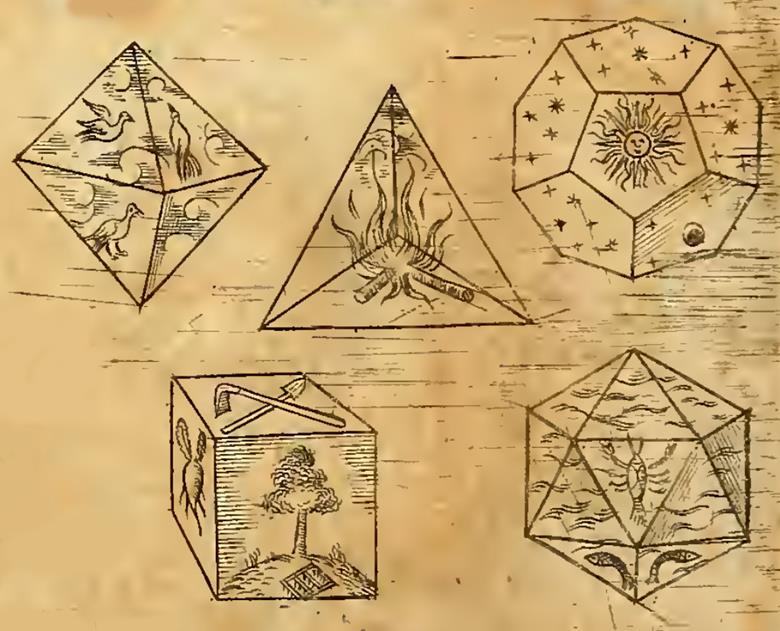Johannes Kepler published the Harmonices Mundi, in 1619 (the page above is an excerpt). It illustrates the five Platonic Solids. Kepler, however, did not discover the Platonic Solids. Plato’s works mention them around 360 B.C. Plato believed each of the Platonic solids was associated with a particular element: fire, water, air, and earth.  Since it has twelve faces, the dodecahedron is associated with the signs of the Zodiac (of which there are also twelve!).

Kepler was fascinated with the construction of these solids and details this in his manuscript. For example, below, check out his breakdown of the icosahedron: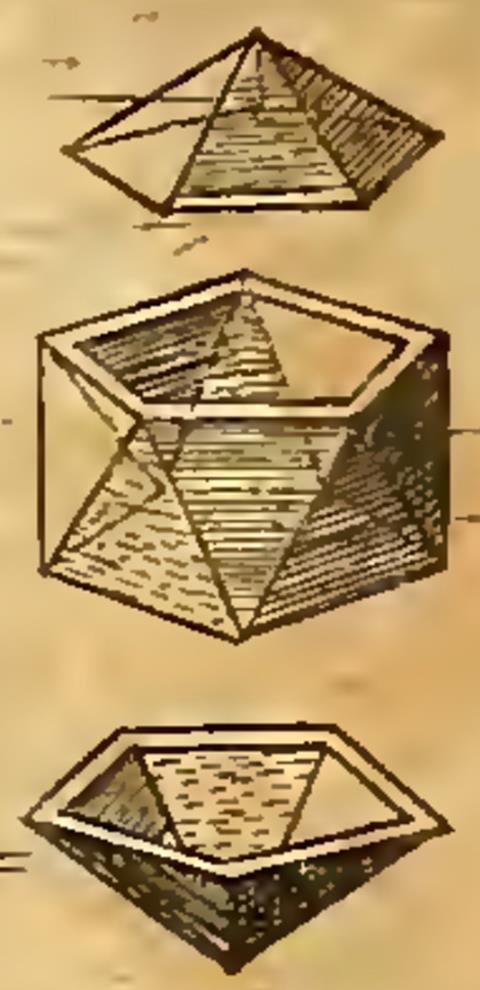Geometiles® allows us to experiment with the construction of these solids just as Kepler did! Below, we can see how we could reconstruct the icosahedron: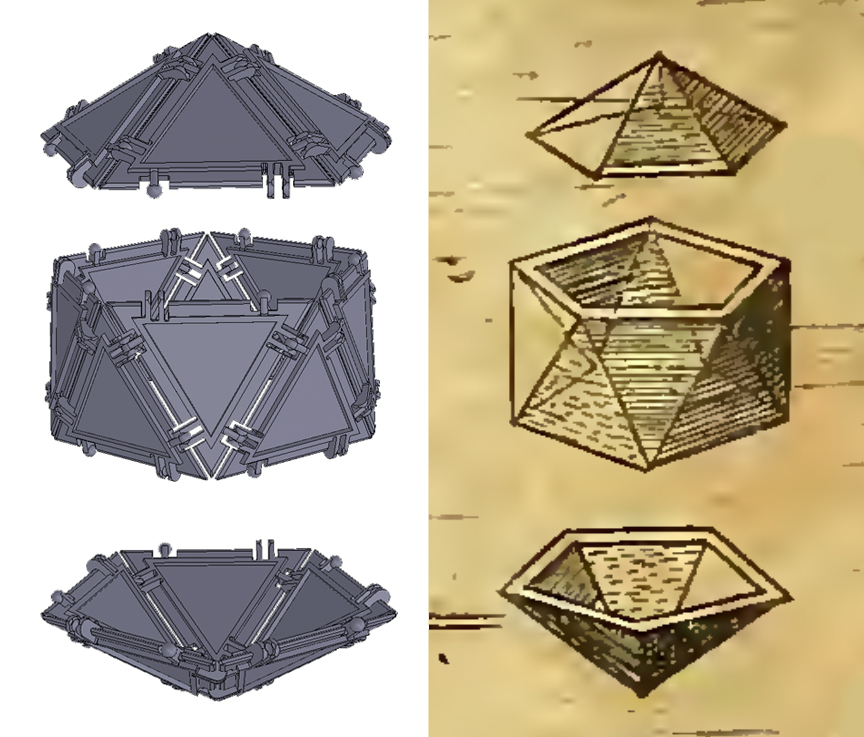Below you can see a modern rendition of the icosahedron I put together with Geometiles®. There is a lot of flexibility here, as I had the option of making each equilateral triangle out of two smaller triangles. Because of this, I could actually create patterns within the classic figure.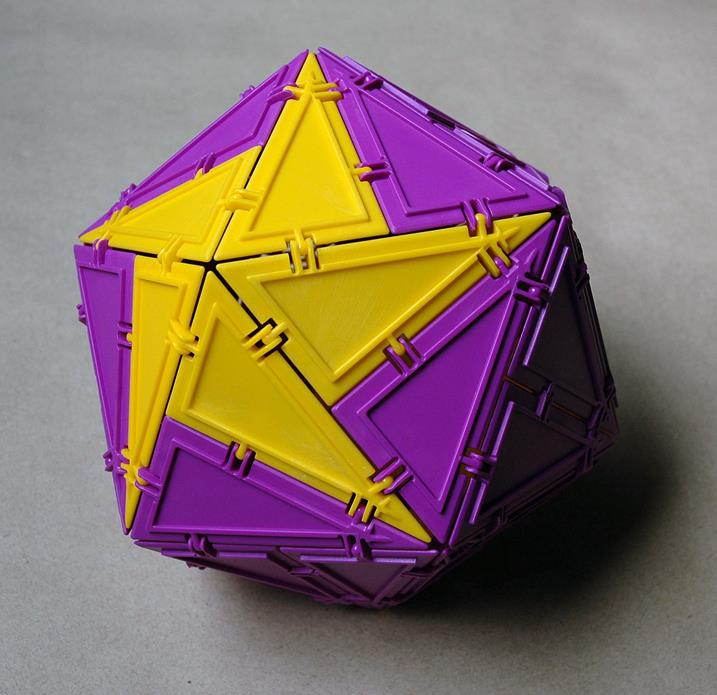Reference for this section: Polyhedra by Peter R. Cromwell, Cambridge University Press, Cambridge, UK 2004.

SaveSave

SaveSave